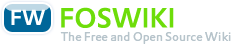# Course Analog Signals and Systems

Responsible: Prof. Dr. Rainer Bartz

## Course

### Meets requirements of following modules(MID)

• in active programs

### Course Organization

Version
created 2013-06-20
VID 4
valid from WS 2012/13
valid to
Course identifiers
Long name Analog Signals and Systems
CID F07_ASS
CEID (exam identifier)

Contact hours per week (SWS)
Lecture 2
Exercise (unsplit)
Exercise (split) 2
Lab
Project
Seminar
Tutorial(voluntary) 1
Total contact hours
Lecture 30
Exercise (unsplit)
Exercise (split) 30
Lab
Project
Seminar
Tutorial (voluntary) 15
Max. capacity
Exercise (unsplit)
Exercise (split) 40
Lab
Project
Seminar

Total effort (hours): 150

• German, 80%
• English, 20%

#### Prerequisites

• all mathematical foundation courses of the program
• trigonometric, exponential and logarithmic functions
• limits, infinite series, partial fraction expansion
• differential and integral calculus
• fundamentals of electrical engineering
• RLC-circuits; complex numbers and functions

• Carlson, G. E.: Signal and Linear System Analysis, John Wiley & Sons, Inc.
• Girod, B.: Einführung in die Systemtheorie, Teubner Verlag
• von Grünigen, D. Ch.: Digitale Signalverarbeitung, Fachbuchverlag Leipzig
• Hsu, H.P.: Signals and Systems, Schaums Outlines
• Meyer, M.: Signalverarbeitung, Verlag Vieweg
• Ohm, J.-R.; Lüke, H. D.: Signalübertragung, Springer-Verlag
• Oppenheim, A.V.; Wilsky, A.S.:Signals & Systems, Prentice Hall
• Werner, M.: Signale und Systeme, Verlag Vieweg

#### Instructors

• Prof. Dr. Rainer Bartz
• Prof. Dr. Harald Elders-Boll
• Prof. Dr. Andreas Lohner

#### Supporting Scientific Staff

• Dipl.-Ing. Martin Seckler
• Dipl.-Ing. Norbert Kellersohn

#### Transcipt Entry

Analog Signals and Systems

### Assessment

Type
wE written exam

Total effort [hours]
wE 10

Frequency: 2-3/year

## Course components

### Lecture/Exercise

#### Objectives

##### Contents
• basic concepts
• signal and system; examples
• classification of signals
• common signals: cos, exp, step, ramp, impulse (Dirac)
• characteristics of signals: symmetry, energy, power, RMS
• odd-even decomposition of signals
• basic operations with signals: time-scaling, time-reversal, time-shift, and their combinations
• characteristics of systems: memory, causality, stability
• block diagrams and their components
• signals
• Fourier series
• Fourier transform (1D) of CT signals
• definition of the Fourier transform
• Fourier transform pairs and theorems; examples
• Parseval's theorem
• auto-correlation function and energy density spectrum
• cross-correlation function
• Laplace transform
• double-sided Laplace transform
• the complex s-plane
• single-sided Laplace transform
• Laplace transform pairs and theorems; examples
• initial and final value theorem
• inverse transform using partial fraction expansion
• relationship to Fourier transform
• sampling
• Fourier transform of impulse train
• ideal sampling
• spectrum of sampled signals
• sampling theorem
• aliasing, examples
• systems; signal transmission
• continuous time (CT) LTI systems
• linear, and time-invariant (LTI) systems
• working with block diagrams
• impulse input and impulse response
• step input and step response
• convolution integral and its evaluation
• determining characteristics of LTI systems: causality, stability
• Bode-plot of the frequency response
• 7 building blocks to construct a Bode-plot
• the transfer function
• pole-zero plot and stability
• design of CT filter systems
• distortionless transmission
• basic filter types: low pass, high pass, band pass, band stop filter

##### Acquired Skills
• students acquire fundamental knowledge on theory and applications of continuous-time signals and systems
• they understand the behavior of typical systems
• they can apply important algorithms for convolution, Fourier-, and Laplace-transform
• they are able to model a system and to analyze it in time and frequency domain
• they can apply system theory to real-world systems (like electrical circuits)

Type
fAP (optional) assessed problem solving
fSP supervised/assisted problem solvingDeutsch English